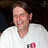# Hammond Transformers

I have a 1959 Magnavox console stereo which fried a power transformer: Its Magnavox
part # 300085-1 and specs:
Pri 117V 1.8A
sec1 560VCT .320 A
sec2 5V 6A
sec3 6.3V 4.4A

do you have something close?

Unfortunately no we do not have a replacement part. I not sure on what to suggest to you how you can go about locate solutions to help you in this repair, we have nothing that is close.

The 370MX datasheet here would seem likely to fit the bill based on specifications, as well as the slightly smaller 370LX. (see datasheet regarding the latter)

Neither is on hand at the moment, but the indicated lead time at the moment (4 weeks) isn’t all that bad, considering current market conditions.

Can I order this direct from you or do I need to go through DigiKey?

Retail sales of Hammond transformer products are done through their authorized distributors, of which Digi-Key is one.

Rick, one last question if you will. The 370MX states that the secondary is NLV. How much voltage loss would happen when connected to the circuit?

I’m not finding specifications for that behavior (sometimes called “regulation”) anywhere for the 370MX. Given that its total output power is approaching 2x that of the original device based on your information however, I’d expect that the 370MX would perform better than the original device in this regard.

Beyond that, tolerances in old tube equipment tended to be sloppier than a two year old eating spaghetti. Even if it was slightly worse, it likely wouldn’t make much difference.

This data sheet @ Hammond:

Indicates that you’ll get 573.1V +/-2% at no load and 550V at 575mA

Decided to go with a #372HX and will reduce the voltage on the 600V line with a resistor. Your thoughts???

I calculated the VA curve (Y=mX+b) for the 370MX.

Let voltage be Y and current be X.
Voltage is 573.1 @ 0 A, therefore the Y-intercept (b) = 573.1
Slope (m) = rise/run =( y2-y1) / (x2-x1) = (573.1-550) / (0-0.575) = 23.1/-0.575 = -40.174

Check results:
-40.174 * 0 + 573.1 = 573.1
-40.174 * 0.575 + 573.1 = 550

Calculate voltage @ 0.32 A
-40.174 * 0.32 + 573.1 = 560.2

560.2 Volts @ 0.32 Amps is almost exactly the output of the original part.

Paul although I do not understand what you said it’s certainly complicated, my only question would be what size of a resistor would I use to drop the 600 volts to 563?

Paul, correction, I ordered the 372HX which has a 600V secondary which I need to reduce to 560VCT.

Dale E. Hinkelman
419-351-4401 cell

Paul although I do not understand what you said it’s certainly complicated, my only question would be what size of a resistor would I use to drop the 600 volts to 563?PaulHutch Trusted Contributor
February 4

I calculated the VA curve (Y=mX+b) for the 370MX.

Let voltage be Y and current be X.
Voltage is 573.1 @ 0 A, therefore the Y-intercept (b) = 573.1
Slope (m) = rise/run = y2-y1/x2-x1 = 573.1-550/0-0.575 = 23.1/-0.575 = -40.174
Check results:
0 * -40.174 + 573.1 = 573.1
0.575 * -40.174 + 573.1 = 550
Calculate voltage @ 0.32 A
0.32 * -40.174 + 573.1 = 560.2

560.2 Volts @ 0.32 Amps is almost exactly the output of the original part.

Sorry you couldn’t follow the math, not sure how I could make it clearer.

Basically when I saw the specs you gave, and the specs for the 370MX, my gut said that it was likely a nearly exact match for the high voltage output at 0.32A. Doing the math showed that yes it is a near perfect match. Although without a no load voltage spec for the original Magnavox transformer, I can’t be certain that the voltage output is a good match at significantly less than the maximum current of 0.32A.

To calculate a resistor to use with the other transformer requires more specifications from the original Magnavox transformer.
Here’s the specs I’d need:

• No load voltage of the high voltage section
• The minimum current draw on the high voltage winding during operation (it will be greater than 0 A)
• The maximum current draw on the high voltage winding during operation (it will be less than 0.32 A)

With that data I could calculate the actual operating voltage target range for the original circuit and attempt to find a resistor value that at least doesn’t burn out the high voltage circuits as the current draw varies in actual use.

Although keep in mind that if the current range variation is high, a fixed resistor may cause a too high voltage variation burning out other portions of the circuit. [Vout = Vin - (A * Ohms)].

Just looked at the 372HX transformer and see that it is only rated for 0.23A on the high voltage winding. That’s a 33% lower than the current rating of the original Magnavox part. I would absolutely NOT recommend the 372HX as a replacement. Wish I’d looked at it before so I could have avoided writing up the last 3 paragraphs.